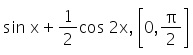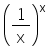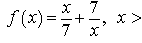# Test: Maxima Minima (Derivative) - 1

## 10 Questions MCQ Test Mathematics (Maths) Class 12 | Test: Maxima Minima (Derivative) - 1

Description
This mock test of Test: Maxima Minima (Derivative) - 1 for JEE helps you for every JEE entrance exam. This contains 10 Multiple Choice Questions for JEE Test: Maxima Minima (Derivative) - 1 (mcq) to study with solutions a complete question bank. The solved questions answers in this Test: Maxima Minima (Derivative) - 1 quiz give you a good mix of easy questions and tough questions. JEE students definitely take this Test: Maxima Minima (Derivative) - 1 exercise for a better result in the exam. You can find other Test: Maxima Minima (Derivative) - 1 extra questions, long questions & short questions for JEE on EduRev as well by searching above.
QUESTION: 1

### The maximum and minimum values of f(x) =are

Solution:

f(x) = sinx + 1/2cos2x
⇒ f'(x) = cos x – sin2x
Now, f'(x) = 0 gives cosx – sin2x = 0
⇒ cos x (1 – 2 sinx) = 0
⇒ cos x = 0, (1 – 2 sinx) = 0
⇒ cos x = 0, sinx = 1/2
⇒ x = π/6 , π/2
Now, f(0) = 1/2,
f(π/6) = 1/2 + 1/4 = 3/4,
f(π/2) = 1 – 1/2 = 1/2
Therefore, the absolute max value = 3/4 and absolute min = 1/2

QUESTION: 2

Solution:
QUESTION: 3

### The maximum value of f (x) = sin x in the interval [π,2π] is​

Solution:

f(x) = sin x
f’(x) =cosx
f”(x) = -sin x
f”(3pi/2) = -sin(3pi/2)
= -(-1)
=> 1 > 0 (local minima)
f(pi) = sin(pi) = 0
f(2pi) = sin(2pi) = 0
Hence, 0 is the maxima.

QUESTION: 4

The maximum and the minimum value of 3x4 – 8x3 + 12x2 – 48x + 1 on the interval [1,4]​

Solution:
QUESTION: 5

The maximum value ofis​

Solution:

For every real number (or) valued function f(x), the values of x which satisfies the equation f1(x)=0 are the point of it's local and global maxima or minima.
This occurs due to the fact that, at the point of maxima or minima, the curve of the function has a zero slope.
We have function f(x) = (1/x)x
We will be using the equation, y = (1/x)x
Taking in both sides we get
ln y = −xlnx
Differentiating both sides with respect to x.y.
dy/dx = −lnx−1
dy/dx =−y(lnx+1)
Equating  dy/dx to 0, we get
−y(lnx+1)=0
Since y is an exponential function it can never be equal to zero, hence
lnx +1 = 0
lnx = −1
x = e(−1)
So, for the maximum value we put x = e^(−1)in f(x) to get the value of f(x) at the point.
f(e^−1) = e(1/e).
Hence the maximum value of the function is (e)1/e

QUESTION: 6

Find the maximum profit that a company can make, if the profit function is given by P(x) = 41 + 24 x – 18x2

Solution:

p’(x) = -24 - 36x
p”(x) = -36
Now, p’(x) = 0  ⇒ x = (-24)/36
x = -⅔
Also, p”(-⅔) = -36 < 0
By the second derivative test,  x = -⅔
Therefore, maximum profit = p(-⅔)
= 41 - 24(-⅔) - 18(-⅔)^2
= 41 +16 - 8
⇒ 49

QUESTION: 7

If f (x) = a log |x| + bx2 + x has extreme values at x = –1 and at x = 2, then values of a and b are

Solution:

f(x) = alog|x| + bx2 + x
f’(x) = a/x + 2bx + 1
f’(-1) = - a - 2b + 1
-a - 2b + 1 = 0
a = 1 - 2b
f’(2) = a/2 + 4b + 1 = 0
a + 8b = -2
Put the value of a in eq(1)
(1 - 2b) + 8b = - 2
6b = -3
b = -½, a = 2

QUESTION: 8

The maximum and minimum values of f (x) = x50-x20 in the interval [0, 1] are

Solution:
QUESTION: 9

Find the maximum and minimum values of f (x) = 2x3 – 24x + 107 in the interval [1, 3].​

Solution:

f(x)=2x³-24x+107 x ∈ [1, 3]
f'(x) = 6x^2 - 24
To find the points equate f'(x) = 0
in closed interval x= -2 doesn't lies,so discard x = -2
now find the value of function at x = 1, 2 ,3
f(1) =2(1)³-24(1)+107
= 2-24+107 = 85
f(2) =2(2)³-24(2)+107
= 16-48+107 = 75
f(3) =2(3)³-24(3)+107
= 54-72+107 = 89
So, the function has maximum value in close interval at x= 3, Maximum value= 89.
minimum value at x= 2, minimum value = 75

QUESTION: 10

Find the point of local maxima or minima for the functionSolution: